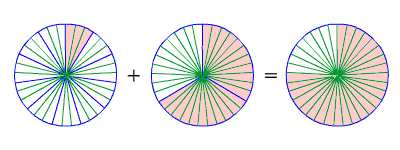When adding and subtracting fractions, the first thing to check is if the denominators are the same.

## Part 1) Adding and Subtracting Fractions with Like Denominators

If the denominators are the same, then it's pretty easy: just add or subtract the numerators , and write the result over the same denominator.

$\frac{2}{13}+\frac{5}{13}=\frac{7}{13}$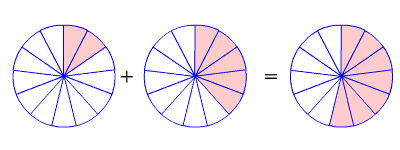$\frac{4}{5}-\frac{1}{5}=\frac{3}{5}$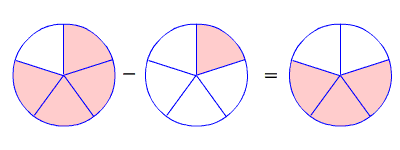You may get an answer which is not in lowest terms , even if the fractions you were adding and subtracting both were. In this case, you have to reduce the fraction .

$\frac{5}{12}+\frac{1}{12}=\frac{6}{12}=\frac{6\text{\hspace{0.17em}}÷\text{\hspace{0.17em}}6}{12\text{\hspace{0.17em}}÷\text{\hspace{0.17em}}6}=\frac{1}{2}$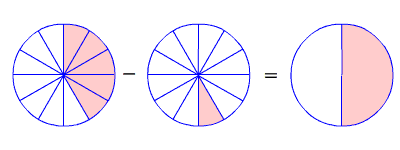## Part 2) Adding and Subtracting Fractions with Unlike Denominators

If the denominators are not the same, then you have to use equivalent fractions which do have a common denominator . To do this, you need to find the least common multiple (LCM) of the two denominators.

For example, suppose you want to add:

$\frac{1}{11}+\frac{2}{3}$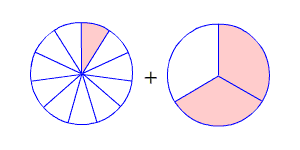The LCM of $3$ and $11$ is $33$ . So, we need to find fractions equivalent to $\frac{1}{11}$ and $\frac{2}{3}$ which have $33$ in the denominator. Multiply the numerator and denominator of $\frac{1}{11}$ by $3$ , and multiply the numerator and denominator of $\frac{2}{3}$ by $11$ .

$\left(\frac{1}{11}×\frac{3}{3}\right)+\left(\frac{2}{3}×\frac{11}{11}\right)=\frac{3}{33}+\frac{22}{33}$

Now we have like denominators, and we can add as described above.

$=\frac{25}{33}$